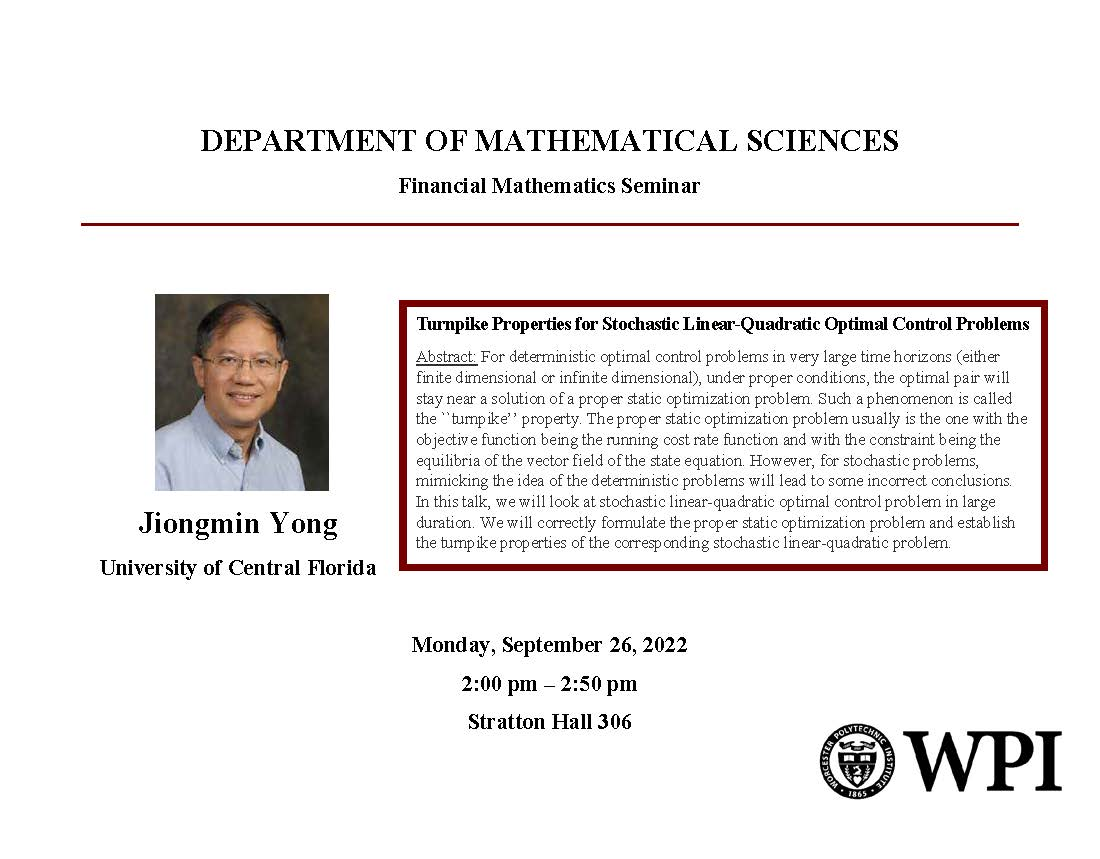# Mathematical Sciences Department, Financial Mathematics Seminar - Jiongmin Yong (University of Central Florida) "Turnpike Properties for Stochastic Linear-Quadratic Optimal Control Problems" SH 306

## Jiongmin Yong 9-26-22.jpgMonday, September 26, 2022
2:00 pm to 2:50 pm

## Location:

Floor/Room #:
306
Mathematical Sciences Department
Financial Mathematics Seminar

Speaker: Jiongmin Yong (UCF)

Monday, September 26, 2022

2:00 pm - 2:50 pm

Stratton Hall 306

Title: Properties for Stochastic Linear-Quadratic Optimal Control Problems

Abstract: For deterministic optimal control problems in very large time horizons (either finite dimensional or infinite dimensional), under proper conditions, the optimal pair will stay near a solution of a proper static optimization problem. Such a phenomenon is called the ``turnpike’’ property. The proper static optimization problem usually is the one with the objective function being the running cost rate function and with the constraint being the equilibria of the vector field of the state equation. However, for stochastic problems, mimicking the idea of the deterministic problems will lead to some incorrect conclusions. In this talk, we will look at stochastic linear-quadratic optimal control problem in large duration. We will correctly formulate the proper static optimization problem and establish the turnpike properties of the corresponding stochastic linear-quadratic problem.

Audience(s):
DEPARTMENT(S):